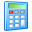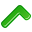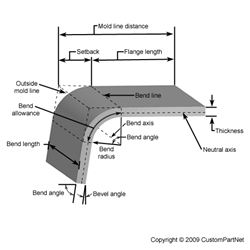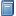Widgets# Bend Allowance Calculator

The bend allowance and bend deduction are two measures that relate the bent length of a piece of sheet metal to the flat length. The bend allowance describes the length of the neutral axis between the bend lines, or in other words, the arc length of the bend. Therefore, the bend allowance added to the flange lengths is equal to the total flat length. The bend deduction, sometimes called the bend compensation, describes how much the outside of the sheet has been stretched. Therefore, the bend deduction equals the difference between the mold line lengths and the total flat length. The mold line lengths are the distances measured to the outside mold line and are equal to the flange lengths plus the setback. The values of the setback, bend allowance, and bend deduction can be calculated from the sheet thickness, bend radius, bend angle, and K-factor. Learn more about Bending.

## Bend Allowance Calculator